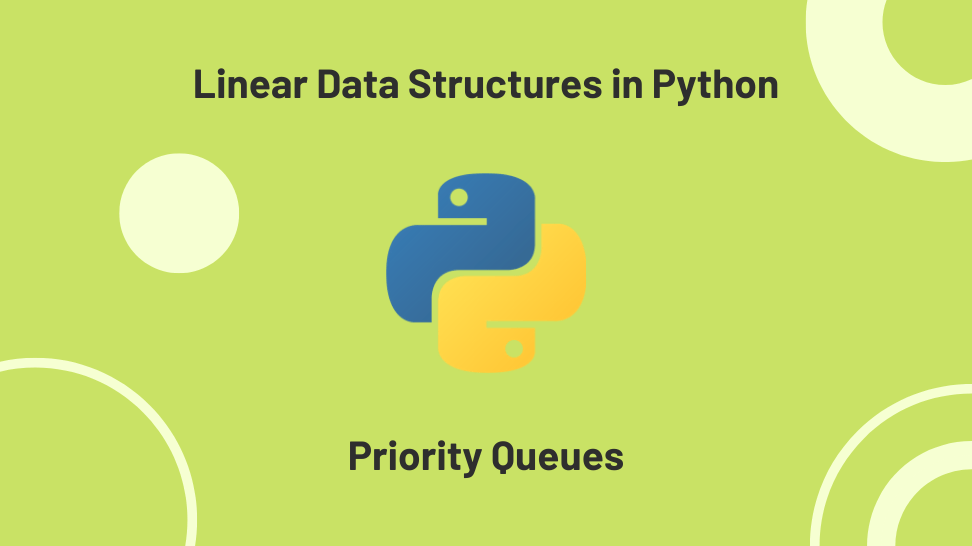In this article, I’ll explore the priority queue, a linear data structure we can easily implement in Python.

This post is the third in a miniseries exploring the implementation of linear data structures. I based the series on my notes while studying for a Python technical interview.

For quick access, here is the list of posts on this series:

1. A Stack Implementation in Python
2. A Queue Implementation in Python
4. Linked Lists Implementations In Python

## Introduction to priority queues

In computer science, a priority queue is an abstract data type in which each element has a priority associated with it. Priority queues differ from queues and stack in the way your retrieve the elements. While the next element in a queue is the first element inserted and in a stack is the last element inserted, in a priority queue, the next element to be retrieve is the one with the highest priority.

There are two types of priority queues:

1. In a min-priority queue, you assign a higher priority to a task with a lower priority number.
2. In a max-priority queue, you assign a higher priority to a task with a higher priority number.

## Uses for a priority queue

Operating systems use priority queues to select the following process to run, load balancing and interrupt handling.

They are also used in bandwidth management to prioritize important data packets.

Certain foundational algorithms, such as Dijkstra’s algorithm, also rely on priority queues.

## Priority queue methods

Most priority queue implementations have the following methods:

2. pq.pull(): returns and removes the highest priority task from the priority queue.
3. pq.peek(): returns the highest priority task from the priority queue.
4. pq.size(): returns the number of task in the priority queue.
5. pq.is_empty(): returns True if the priority queue is empty.

## Priority queue implementation

We’ll use the heapq available from the Lib/heapq.py module in Python for our priority queue implementation. The reason for using heapq instead of other data types is that you can push and pop in O(log n) time while keeping the underlying data in order of priority.

Let’s go over our priority queue implementation.

### The PriorityQueue class

We define a PriorityQueue class with a constructor that instantiates an empty list and a __str__ method that returns a readable representation of the priority queue object. The class has an insertion_count attribute. We use this attribute to keep track of the insertion order. At the top of the module, we must import heapq:

### pq.is_empty():

The is_empty method returns True if the priority queue is empty.

This method has a constant time complexity O(1).

The insert method adds an item to the priority queue, using the heappush method from heapq. The item added is a tuple that contains the priority, the insertion count, and the task.

We increase the insertion count after every insert. We use the insertion count to decide which task to return when several tasks have the same priority.

This method has a logarithmic time complexity O(log n).

### pq.pull():

The pull method returns and removes the task with the highest priority using the heappop method from heapq. To avoid getting an IndexError when pulling from an empty priority queue, we first check if it is empty and return None.

This method has a logarithmic time complexity O(log n).

### pq.peek()

The peek method returns the task with the highest priority. As with pull, we need to return None if the priority queue is empty to avoid an IndexError.

This method has a constant time complexity O(1).

### pq.size()

The size method returns the length of the priority queue.

This method has a constant time complexity O(1).

### The full implementation

Below is the full implementation and some test cases:

## Conclusion

In this article, we used a heapq to implement a priority queue. Our priority queue returns the task with the highest priority first. In cases where several tasks have the same priority, they are returned in the order of insertion.

In the next article, we’ll explore linked lists. See you there!

## References

Wikipedia – Priority queue

Baeldung – Priority queue

Python Documentation – heapq – Heap queue algorithm

Stackoverflow – What is the time complexity of functions in the heapq library?